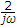# Electronics and Communication Engineering - Signals and Systems

Exercise : Signals and Systems - Section 9
1.
The total area under the probability distribution curve is
1
0
depend upon curve equation
Explanation:
No answer description is available. Let's discuss.

2.
Which one of following is correct condition to check the stability of system?
Bounded I/P unbounded O/P
Bounded I/P and O/P
Bounded O/P and I/P
Bounded I/P Bounded O/P
Explanation:
No answer description is available. Let's discuss.

3.
The inverse Fourier transform of the function F(ω) =is
sin ωt
cos ωt
sgnt
u(t)
Explanation:
No answer description is available. Let's discuss.

4.
Let f1(t) = G1(t) + 4, f2(t) = G2(t) + 3. If G1(t) and G2(t) are uncorrected then the correlation between f1(t) and f2(t) are
12
7
6
zero